## A gentle (and short) introduction to Gröbner Bases

Taken from my report for a Computer Algebra course.

## Motivation

We know there are plenty of methods to solve a system of linear equations (to name a few: Gauss elimination, QR or LU factorization). In fact, it is straightforward to check whether a linear system has any solutions, and if it does, how many of them there are. But what if the system is made of non-linear equations? The invention of Groebner bases and the field of computational algebra came up to answer these questions.

In this text we will recap the theory behind single-variable polynomials and extend it to multiple-variable ones, ultimately getting to the definition of Groebner bases.

In some cases, the transition from one to multiple variables is smooth and pretty much an extension of the simple case (for example for the Greatest Common Divisor algorithm). In other cases, however, there are conceptual jumps to be made. To give an example, single variable polynomials have always a finite number of roots, while this does not hold for multivariable polynomials. Intuitively, the reason is that a polynomial in one variable describes a curve in the plane, which can only intersect the x-axis a discrete and finite number of times. On the other hand, a multivariate polynomial describes a surface in space, which will always intersect the 0-plane in a continuum of points.

## But WHY is the Lattices Bounded Distance Decoding Problem difficult?

This is taken from my Master Thesis on Homomorphic Signatures over Lattices.

### Introduction to lattices and the Bounded Distance Decoding Problem

A lattice is a discrete subgroup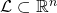, where the word discrete means that each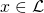has a neighborhood in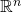that, when intersected with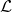results initself only. One can think of lattices as being grids, although the coordinates of the points need not be integer. Indeed, all lattices are isomorphic to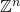, but it may be a grid of points with non-integer coordinates.Another very nice way to define a lattice is: givenindependent vectors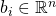, the latticegenerated by that base is the set of all linear combinations of them with integer coefficients: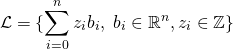Then, we can go on to define the Bounded Distance Decoding problem (BDD), which is used in lattice-based cryptography (more specifically, for example in trapdoor homomorphic encryption) and believed to be hard in general.

Given an arbitrary basis of a lattice, and a pointnot necessarily belonging to, find the point ofthat is closest to. We are also guaranteed thatis very close to one of the lattice points. Notice how we are relying on an arbitrary basis – if we claim to be able to solve the problem, we should be able to do so with any basis.

Now, as the literature goes, this is a problem that is hard in general, but easy if the basis is nice enough. So, for example for encryption, the idea is that we can encode our secret message as a lattice point, and then add to it some small noise (i.e. a small element). This basically generates an instance of the BDD problem, and then the decoding can only be done by someone who holds the good basis for the lattice, while those having a bad basis are going to have a hard time decrypting the ciphertext.

However, albeit of course there is no proof of this (it is a problem believed to be hard), I wanted to get at least some clue on why it should be easy with a nice basis and hard with a bad one (GGH is an example schema that employs techniques based on this).

So now to our real question. But WHY is the Bounded Distance Decoding problem hard (or easy)?

## Relationship between reduced rings, radical ideals and nilpotent elements

This post aims at providing some intuition and meaning for the following algebra relationship:

Reduced ring – Radical ideal – Nilpotent

## Reduced ring – Radical ideal – Nilpotent

A basic fact of ring theory is that if you take a ring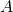and quotient it for a (double-sided) radical idealyou get a reduced ring. Let us suppose A is a commutative ring and understand why this fact is true.

Nilpotent element
Def.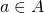is nilpotentInformally, a nilpotent element is like a road ending in the middle of nowhere, collapsing in the depth of an abyss. You are driving on it, following the powers of, and then all of a sudden, with no explanation, your road ends in a big black hole. Indeed, the zero really acts as some kind of black hole, attracting nilpotent-made roads at some point or another: we can think of nilpotent roads as spiraling into the zero.